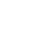# Python达到函数的尾部时仍然没有遇到语句，就会自动返回None

``def my_function(x, y, z=1.5):  ``if z > 1:  ``    return z * (x + y)  ``else:  ``    return z / (x + y)``

``my_function(5, 6, z=0.7)  ``my_function(3.14, 7, 3.5)  ``my_function(10, 20)``

``my_function(x=5, y=6, z=7)  ``my_function(y=6, x=5, z=7)``

01 命名空间、作用域和本地函数

``def func():  ``    a = []  ``    for i in range(5):  ``        a.append(i)``

``a = []  ``def func():  ``    for i in range(5):  ``        a.append(i)``

``In : a = None  ``In : def bind_a_variable():  ``.....:        global a  ``.....:        a = []  ``.....:        bind_a_variable()  ``.....:  ``In : print(a)  ``[]``

02 返回多个值

``def f():  ``a = 5  ``b = 6  ``c = 7  ``return a, b, c  ``a, b, c = f()``

``return_value = f()``

``def f():  ``a = 5  ``b = 6  ``c = 7  ``return {'a' : a, 'b' : b, 'c' : c}  ``

03 函数是对象

``In : states = [' Alabama ', 'Georgia!', 'Georgia', 'georgia', 'FlOrIda',  ``.....: 'south carolina##', 'West virginia?']``

``import re  ``def clean_strings(strings):  ``    result = []  ``    for value in strings:  ``        value = value.strip()  ``        value = re.sub('[!#?]', '', value)  ``        value = value.title()  ``        result.append(value)  ``    return result``

``In : clean_strings(states)  ``Out:  ``['Alabama',  ``'Georgia',  ``'Georgia',  ``'Georgia',  ``'Florida',  ``'South Carolina',  ``'West Virginia']``

``def remove_punctuation(value):  ``return re.sub('[!#?]', '', value)  ``clean_ops = [str.strip, remove_punctuation, str.title]  ``def clean_strings(strings, ops):  ``    result = []  ``    for value in strings:  ``        for function in ops:  ``            value = function(value)  ``        result.append(value)  ``    return result``

``In : clean_strings(states, clean_ops)  ``Out:  ``['Alabama',  ``'Georgia',  ``'Georgia',  ``'Georgia',  ``'Florida',  ``'South Carolina',  ``'West Virginia']``

``In : for x in map(remove_punctuation, states):  ``.....:        print(x)  ``Alabama  ``Georgia  ``Georgia  ``georgia  ``FlOrIda  ``south carolina  ``West virginia``

04 匿名(Lambda)函数

Python支持所谓的匿名或lambda函数。匿名函数是一种通过单个语句生成函数的方式，其结果是返回值。匿名函数使用lambda关键字定义，该关键字仅表达“我们声明一个匿名函数”的意思：

``def short_function(x):  ``    return x * 2  ``equiv_anon = lambda x: x * 2  ``

``def apply_to_list(some_list, f):  ``    return [f(x) for x in some_list]  ``ints = [4, 0, 1, 5, 6]  ``apply_to_list(ints, lambda x: x * 2)``

``In : strings = ['foo', 'card', 'bar', 'aaaa', 'abab']``

``In : strings.sort(key=lambda x: len(set(list(x))))  ``In : strings  ``Out: ['aaaa', 'foo', 'abab', 'bar', 'card']``

05 柯里化：部分函数应用

``def add_numbers(x, y):  ``return x + y``

``add_five = lambda y: add_numbers(5, y)``

``from functools import partial  ``add_five = partial(add_numbers, 5)``

06 生成器

``In : some_dict = {'a': 1, 'b': 2, 'c': 3}  ``In : for key in some_dict:  ``.....:        print(key)  ``a   ``b  ``c  ``

``In : dict_iterator = iter(some_dict)  ``In : dict_iterator  ``Out: ``

``In : list(dict_iterator)  ``Out: ['a', 'b', 'c']``

``def squares(n=10):  ``print('Generating squares from 1 to {0}'.format(n ** 2))  ``for i in range(1, n + 1):  ``    yield i ** 2  ``

``In : gen = squares()  ``In : gen  ``Out:   ``

``In : for x in gen:  ``.....: print(x, end=' ')  ``Generating squares from 1 to 100  ``1 4 9 16 25 36 49 64 81 100  ``

1. 生成器表达式

``In : gen = (x ** 2 for x in range(100))  ``In : gen  ``Out:  at 0x7fbbd5ab29e8>  ``

``def _make_gen():  ``for x in range(100):  ``    yield x ** 2  ``gen = _make_gen()  ``

``In : sum(x ** 2 for x in range(100))  ``Out: 328350  ``In : dict((i, i **2) for i in range(5))  ``Out: {0: 0, 1: 1, 2: 4, 3: 9, 4: 16}  ``

2. itertools模块

``In : import itertools  ``In : first_letter = lambda x: x  ``In : names = ['Alan', 'Adam', 'Wes', 'Will', 'Albert', 'Steven']  ``In : for letter, names in itertools.groupby(names, first_letter):  ``.....:        print(letter, list(names)) # names是一个生成器  ``A ['Alan', 'Adam']  ``W ['Wes', 'Will']  ``A ['Albert']  ``S ['Steven']  ``

combinations(iterable, k)

permutations(iterable, k)

groupby(iterable[, keyfunc])

product(*iterables, repeat=1)

07 错误和异常处理

``In : float('1.2345')  ``Out: 1.2345  ``In : float('something')  ``---------------------------------------------------------------------------  ``ValueError Traceback (most recent call last)  ``input-198-439904410854> in <module>()  ``----> 1 float('something')  ``ValueError: could not convert string to float: 'something' ``

``def attempt_float(x):  ``try:  ``    return float(x)  ``except:  ``    return x  ``

``In : attempt_float('1.2345')  ``Out: 1.2345  ``In : attempt_float('something')  ``Out: 'something  ``

``In : float((1, 2))  ``---------------------------------------------------------------------------  ``TypeError Traceback (most recent call last)  ``input-202-842079ebb635> in <module>()  ``----> 1 float((1, 2))  ``TypeError: float() argument must be a string or a number, not 'tuple'  ``

``def attempt_float(x):  ``try:  ``    return float(x)  ``except ValueError:  ``    return x  ``

``In : attempt_float((1, 2))  ``---------------------------------------------------------------------------  ``TypeError Traceback (most recent call last)  ``input-204-9bdfd730cead> in <module>()  ``----> 1 attempt_float((1, 2))  ``input-203-3e06b8379b6b> in attempt_float(x)  ``1 def attempt_float(x):  ``2 try:  ``----> 3 return float(x)  ``4 except ValueError:  ``5 return x  ``TypeError: float() argument must be a string or a number, not 'tuple'  ``

``def attempt_float(x):  ``try:  ``    return float(x)  ``except (TypeError, ValueError):  ``    return x  ``

``f = open(path, 'w')  ``try:  ``    write_to_file(f)  ``finally:  ``    f.close()  ``

``f = open(path, 'w')  ``try:  ``    write_to_file(f)  ``except:  ``    print('Failed')  ``else:  ``    print('Succeeded')  ``finally:  ``    f.close()  ``

IPython中的异常

``In : %run examples/ipython_bug.py  ``---------------------------------------------------------------------------  ``AssertionError Traceback (most recent call last)  ``/home/wesm/code/pydata-book/examples/ipython_bug.py in ()  ``13 throws_an_exception()  ``14  ``---> 15 calling_things()  ``/home/wesm/code/pydata-book/examples/ipython_bug.py in calling_things()  ``11 def calling_things():  ``12 works_fine()  ``---> 13 throws_an_exception()  ``14  ``15 calling_things()  ``/home/wesm/code/pydata-book/examples/ipython_bug.py in throws_an_exception()  ``7 a = 5  ``8 b = 6  ``----> 9 assert(a + b == 10)  ``10  ``11 def calling_things():  ``AssertionError:  ``《Python达到函数的尾部时仍然没有遇到语句，就会自动返回None》